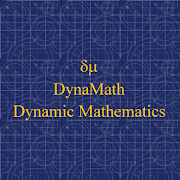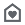# DynaMathEveryone
This application displays unlimited number of completely worked out examples in the following topics:

01. Greatest Common Divisor
02. Least Common Multiple
03. Fractions
04. Properties of Exponents 1
05. Properties of Exponents 2
06. Properties of Exponents 3
07. Properties of Exponents 4
08. Properties of Exponents 5
09. Applications of Properties of Exponents
10 F.O.I.L. Method
11. Expand (A + B) ^2
12. Expand (A - B)^2
13. Expand (A + B)(A - B)
14. Expand (A + B)^3
15. Expand (A - B )^3
16. Expand (A + B)(A^2 - AB + B^2)
17. Expand (A - B)(A^2 + AB + B^2)
18. Factor A^2 + 2AB + B^2
19. Factor A^2 - 2AB + B^2
20. Factor A^2 - B^2
21. Factor A^3 + 3A^2B + 3AB^2 + B^3
22. Factor A^3 - 3A^2B + 3AB^2 - B^3
23. Factor A^3 + B^3
24. Factor A^3 - B^3
25. Simple Factoring
26. Group Factoring
27. Trinomial Factoring
29. Multiply Polynomials
30. Long Division
31. Synthetic Division
32. Slope Intercept Line Equation
33. Point Slope Line Equation
34. Point Point Line Equation
35. Point of Intersection
36. Mid Point I
37. Mid Point II
38. Distance between 2 points
39. If 3 points colinear
40. If 3 points form a right angle triangle
41. Equation of circle given center and radius
42. Find center and radius of circle
43. Equation of circle given its diameter
44. Ellipse
45. Hyperbola
46. Solve Linear Equation
48. Solve Quadratic Equation (Completing the square)
50. Solve Quadratic Equation (Imaginery Solutions)
52. Multiply/Divide Rational Expressions
53. Polynomial with real zeroes
54. Complex Numbers
55. Complex Zeroes
56. Logs
57. Differentiation (20+ routines)
58. Integration (30+ routines)
59. Normal Distribution
Collapse

Review Policy

## What's New

Version 1.1.1

1. App name has been changed to DynaMath for Dynamic Mathematics.

2. The background color has been changed to a less bright color from white. The text color has been changed to light black from blue.

3. The app launcher icon has been changed so as the promotional graphics.The new icon contains the lowere case Greek letters delta and mu for DynaMath (DM).

Version 1.1.2

1. 30+ integral calculus routines were lumped together. Now they have been separated into individual routines.
Collapse

Eligible for Family LibraryEligible if bought after 7/2/2016. Learn More
Updated
August 8, 2013
Size
1.3M
Installs
50+
Current Version
1.1.2
Requires Android
2.1 and up
Content Rating
Everyone
Permissions
Offered By
JDS Plugins
Developer
JDS Front Street Jessup, PA 18434